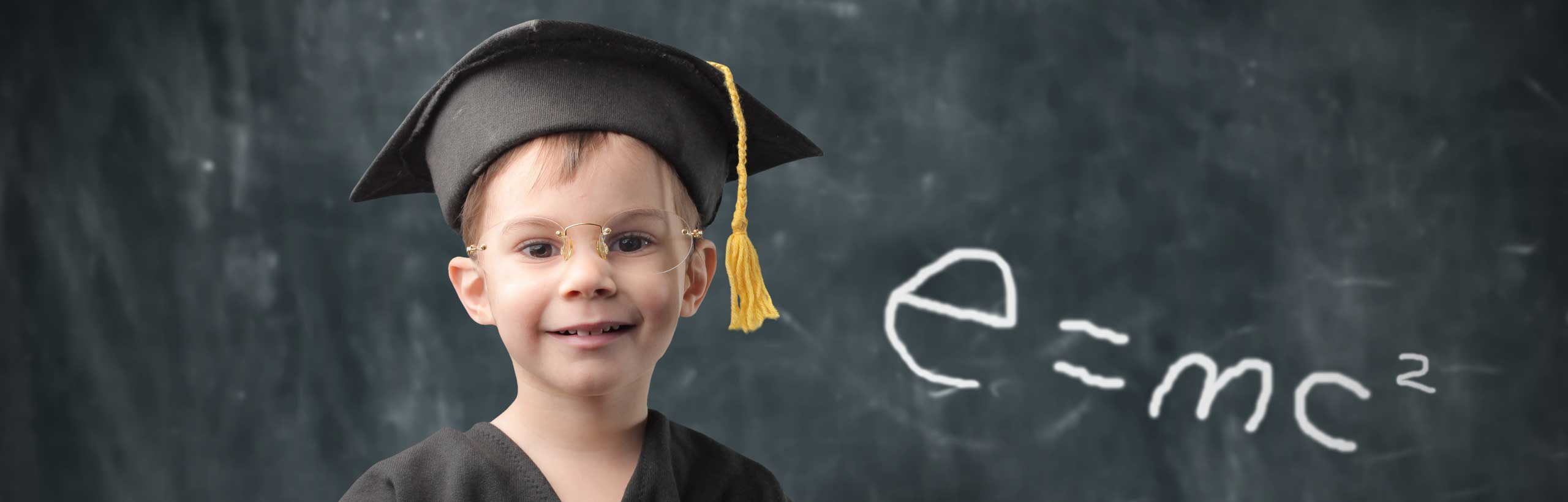Cone Calculator

An easy to use online calculator to calculate the lateral surface area and the volume of a right cone given any two of its dimensions: radius r, height h and slant height s. Another calculator to make a cone from a sector is included in this site.Formulas of Area and Volume of a Cone Used in this Calculator

Let r be the radius of the base and h the height of the cone as shown in the above diagram. The area of the surface and volume of the cone are given by:
Base surface area $$B_a= \pi r^2$$ (area of the circular base)
Lateral surface area: $$L_a = \pi r \sqrt{r^2+h^2}$$ (area of lateral surface)
Total surface area $$T_a= \pi r^2 + \pi r \sqrt{r^2+h^2}$$
Volume $$= \dfrac{1}{3} \pi r^2 h$$
Slant height s $$= \sqrt{r^2+h^2}$$ (length from the apex of the cone to a point on the circle making the base)
Height h $$= \sqrt{h^2-r^2}$$
Radius r $$= \sqrt{h^2-h^2}$$

Use the Calculator to Find the Area and Volume of the Cone Given its Height h and Radius r

Enter the radius r of the base and height h of the cone as positive real numbers and press "Calculate Area and Volume". The outputs are the lateral surface area $$L_a$$ , the base area $$B_a$$, the total area $$T_a$$, the volume of the cone V and the slant height s.

 Radius r = 5 Height h = 15 Lateral Area $$L_a$$ = Base Area $$B_a$$ = Total Area $$T_a$$ = Volume V = Slant Height s =

Use the Calculator to Find the Area and the Volume of the Cone Given its Radius r and Slant Height s

Enter the radius r of the base and the slant height s of the cone as positive real numbers such that s is greater than r and then press "Calculate Area and Volume". The outputs are the lateral surface area $$L_a$$ , the base area $$B_a$$, the total area $$T_a$$, the volume of the cone V and the height h.

 Radius r = 10 Slant Height s = 20 Lateral Area $$L_a$$ = Base Area $$B_a$$ = Total Area $$T_a$$ = Volume V = Height h =

Use the Calculator to Find the Area and the Volume of the Cone Given its Height h and Slant Height s

Enter the height h and the slant height s of the cone as positive real numbers such that s greater then h and press "Calculate Area and Volume". The outputs are the lateral surface area $$L_a$$ , the base area $$B_a$$, the total area $$T_a$$, the volume of the cone V and the radius r.

 Height h = 8 Slant Height s = 12 Lateral Area $$L_a$$ = Base Area $$B_a$$ = Total Area $$T_a$$ = Volume V = Radius r =